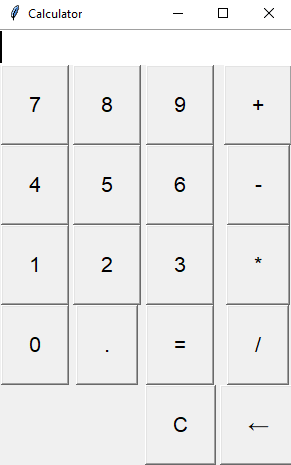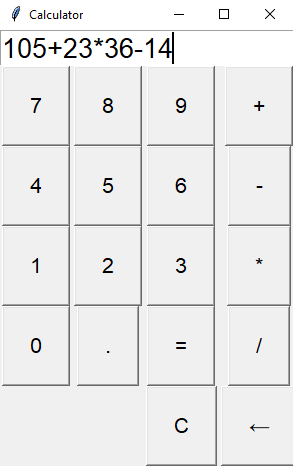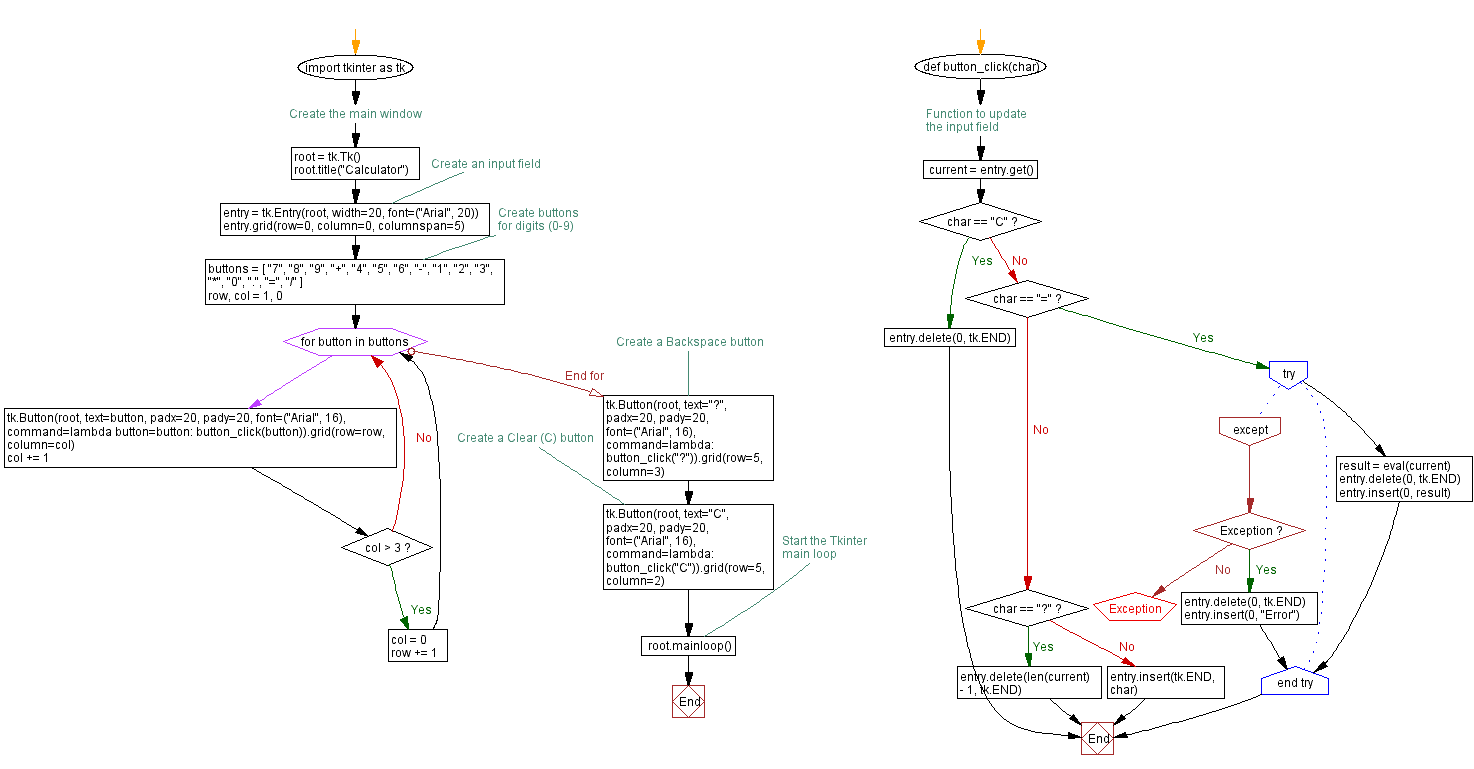﻿ Python Tkinter simple calculator example

# Python Tkinter simple calculator example

## Python Tkinter Events and Event Handling: Exercise-3 with Solution

Write a Python program that implements a simple calculator application using Tkinter with buttons for digits (0-9) and arithmetic operators (+, -, *, /). Implement event handling to calculate.

Sample Solution:

Python Code:

``````import tkinter as tk
# Function to update the input field
def button_click(char):
current = entry.get()
if char == "C":
entry.delete(0, tk.END)
elif char == "=":
try:
result = eval(current)
entry.delete(0, tk.END)
entry.insert(0, result)
except Exception:
entry.delete(0, tk.END)
entry.insert(0, "Error")
elif char == "←":
entry.delete(len(current) - 1, tk.END)
else:
entry.insert(tk.END, char)

# Create the main window
root = tk.Tk()
root.title("Calculator")

# Create an input field
entry = tk.Entry(root, width=20, font=("Arial", 20))
entry.grid(row=0, column=0, columnspan=5)

# Create buttons for digits (0-9)
buttons = [
"7", "8", "9", "+",
"4", "5", "6", "-",
"1", "2", "3", "*",
"0", ".", "=", "/"
]
row, col = 1, 0
for button in buttons:
command=lambda button=button: button_click(button)).grid(row=row, column=col)
col += 1
if col > 3:
col = 0
row += 1

# Create a Backspace button
command=lambda: button_click("←")).grid(row=5, column=3)

# Create a Clear (C) button
command=lambda: button_click("C")).grid(row=5, column=2)

# Start the Tkinter main loop
root.mainloop()
```
```

Explanation:

In the exercise above -

• Import the "tkinter" module.
• Define functions "button_click(), "clear", and calculate for handling button clicks.
• Create the main Tkinter window root and set its title.
• Create an input field using 'tk.Entry' for displaying and entering expressions.
• Create buttons for digits (0-9) and operators (+, -, *, /) using tk.Button, and we bind the button_click function to each button to update the input field.
• Create Clear (C), Equals (=) buttons and add a Backspace (←) button that, when clicked, deletes the last character from the input field. We bind the clear and calculate functions to these buttons.
• Finally, start the Tkinter main loop with root.mainloop().

Output:

``````
``````

Flowchart:Python Code Editor:

What is the difficulty level of this exercise?

Test your Programming skills with w3resource's quiz.

﻿

## Python: Tips of the Day

Summing a sequence of numbers (calculating the sum of zero to ten with skips):

```>>> l = range(0,10,2)
>>> sum(l)
20
```

We are closing our Disqus commenting system for some maintenanace issues. You may write to us at reach[at]yahoo[dot]com or visit us at Facebook